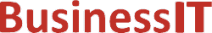O nás     Inzerce     KontaktSpolehlivé informace o IT již od roku 2011[Linux manuál]

# drem, dremf, dreml, remainder, remainderf, remainderl: funkce s pohyblivou řádovou čárkou

Originální popis anglicky: drem, dremf, dreml, remainder, remainderf, remainderl - floating-point remainder function

Návod, kniha: Linux Programmer's Manual

## STRUČNĚ

```#include <math.h>

/* The C99 versions */
double remainder(double x, double y);
float remainderf(float x, float y);
long double remainderl(long double x, long double y);

/* Obsolete synonyms */
double drem(double x, double y);
float dremf(float x, float y);
long double dreml(long double x, long double y);

```

## POPIS / INSTRUKCE

The remainder() function computes the remainder of dividing x by y. The return value is x - n * y, where n is the value x / y, rounded to the nearest integer. If this quotient is 1/2 (mod 1), it is rounded to the nearest even number (independent of the current rounding mode). If the return value is 0, it has the sign of x.
The drem() function does precisely the same thing.

## NÁVRATOVÁ HODNOTA

The remainder() function returns the remainder, unless y is zero, when the function fails and errno is set.

## CHYBY / ERRORY

EDOM
The denominator y is zero.

## ODPOVÍDAJÍCÍ

IEC 60559. The three remainder*() functions are from C99. The function drem() is from BSD 4.3. The float and long double variants dremf() and dreml() exist on some systems, such as Tru64 and glibc2.

## EXAMPLE

The call "remainder(29.0, 3.0)" returns -1.

## SOUVISEJÍCÍ

fmod(3)
 2003-11-18
©2011-2020 BusinessIT.cz, ISSN 1805-0522 | Názvy použité v textech mohou být ochrannými známkami příslušných vlastníků.
Provozovatel: Bispiral, s.r.o., kontakt: BusinessIT(at)Bispiral.com | Inzerce: Best Online Media, s.r.o., zuzana@online-media.cz
O vydavateli | Pravidla webu BusinessIT.cz a ochrana soukromí | pg(8062)